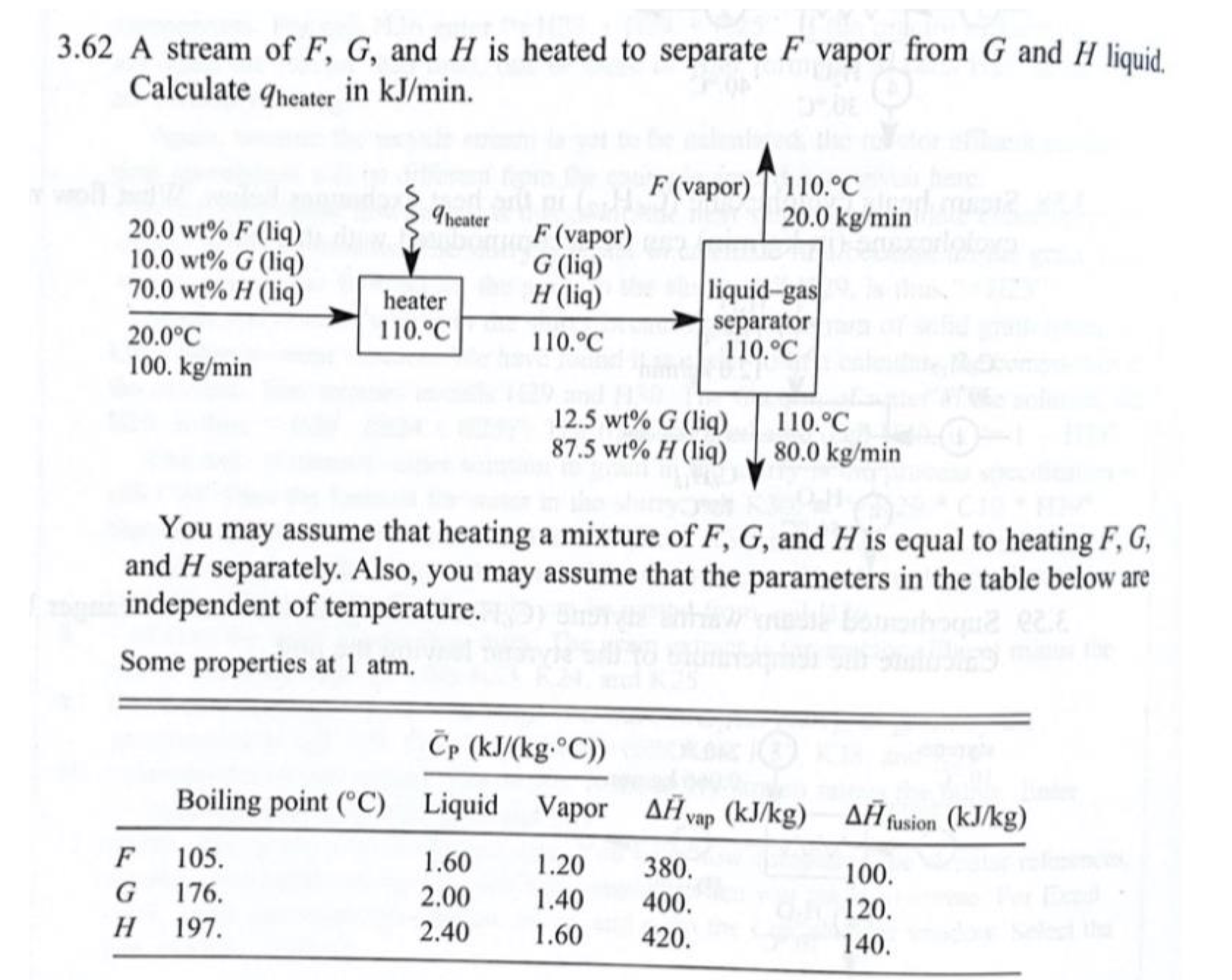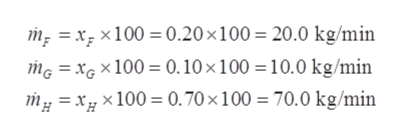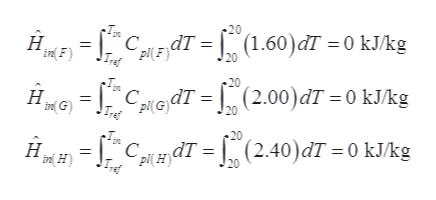# 3.62 A stream of F, G, and H is heated to separate F vapor from G and H liquid.Calculate qheater in kJ/min.F (vapor) T 110.°C20.0 kg/minIheater20.0 wt% F (liq)10.0 wt% G (liq)70.0 wt% H (liq)F (vapor)G (liq)H (liq)liquid-gasseparator110.°Cheater110.°C20.0°C110.°C100. kg/min12.5 wt% G (liq)87.5 wt% H (liq)110.°C80.0 kg/minYou may assume that heating a mixture of F, G, and H is equal to heating F, G,and H separately. Also, you may assume that the parameters in the table below areindependent of temperature.Some properties at 1 atm.Čp (kJ/(kg.°C))Boiling point (°C) Liquid Vapor AĤvap (kJ/kg) AĤfusion (kJ/kg)105.1.601.20380.100.176.2.001.40400.120.Н197.2.401.60420.140.

Question
7 viewshelp_outlineImage Transcriptionclose3.62 A stream of F, G, and H is heated to separate F vapor from G and H liquid. Calculate qheater in kJ/min. F (vapor) T 110.°C 20.0 kg/min Iheater 20.0 wt% F (liq) 10.0 wt% G (liq) 70.0 wt% H (liq) F (vapor) G (liq) H (liq) liquid-gas separator 110.°C heater 110.°C 20.0°C 110.°C 100. kg/min 12.5 wt% G (liq) 87.5 wt% H (liq) 110.°C 80.0 kg/min You may assume that heating a mixture of F, G, and H is equal to heating F, G, and H separately. Also, you may assume that the parameters in the table below are independent of temperature. Some properties at 1 atm. Čp (kJ/(kg.°C)) Boiling point (°C) Liquid Vapor AĤvap (kJ/kg) AĤfusion (kJ/kg) 105. 1.60 1.20 380. 100. 176. 2.00 1.40 400. 120. Н 197. 2.40 1.60 420. 140. fullscreen
check_circle

Step 1

The basis of the calculation is taken as 100.0 kg/min of the feed entering the heater.

Mass fraction of F, G, and H are:

xF = 0.20

xG = 0.10

xH = 0.70

Mass flowrate of each of the components (F, G, and H) will thus be,

Note that he mass flowrate of each of the components is same in inlet and outlet to the heater.help_outlineImage Transcriptionclosem; = x; ×100 = 0.20×100= 20.0 kg/min mg = xG × 100 = 0.10 ×100 =10.0 kg/min my = xg x100 = 0.70×100 = 70.0 kg/min fullscreen
Step 2

Kinetic and potential energy of the system is neglected along with any shaft work which is also neglected. Apply energy balance around the heater as:

Step 3

Reference temperature, Tref for the given process is taken as 20 ̊C.

For component F, heat of vaporization will be considered as its state changes fr...help_outlineImage Transcriptionclose(1.60)đT = 0 kJ/kg Н. in F) piLEdT = ] Iref CledT = [ (2.00)dT = 0 kJ/kg Hgo = н. in G) Clen dT = [° (2.40)đT = 0 kJ/kg I (p(H) ind H) fullscreen

### Want to see the full answer?

See Solution

#### Want to see this answer and more?

Solutions are written by subject experts who are available 24/7. Questions are typically answered within 1 hour.*

See Solution
*Response times may vary by subject and question.
Tagged in